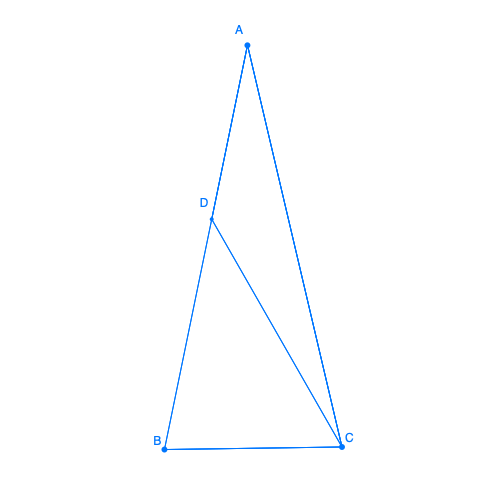Geometry - Mac OS App

# Exercises

The sketches can be opened with the Geometry app, so that you can move the dots around and rearrange the problem's drawing.

## Easy

1.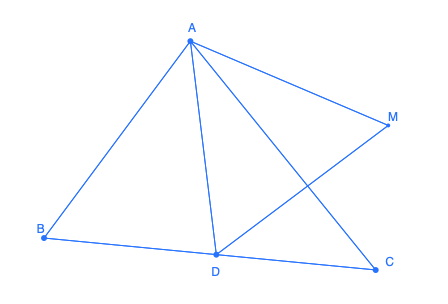In a triangle ABC, find the set of points M for which there exists a point D on line segment [BC] so that triangle BMD is equilateral.

2.In triangle ABC, the line D parallel to (BC) intersects [AB] in B' and [AC] in C'. The lines (BC') and (CB') intersect in M. Find the set of points M when D varies.

3.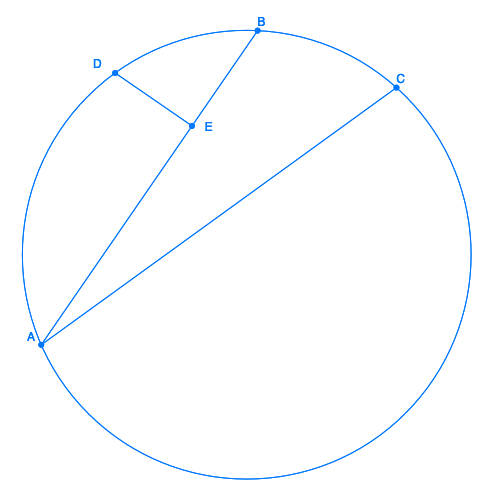Let AB and AC be two arcs of a cercle. We assume that the length AB is strictly greater than BC. Let D be the middle of the arc AC and E be its orthogonal projection on [AB].
Show that AE = EB + EC.

N.B: This theorem is due to Archimede.

## Medium

1.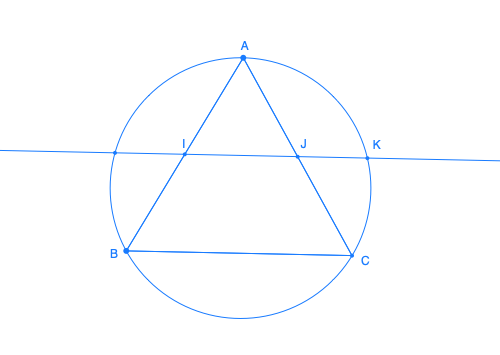In the equilateral triangle ABC, the line that passes by the middle I and J of [AB] and [AC] intersect in K the (small) arc (AC of the circle circumscribed to ABC. Show that IK/IJ = IJ/JK and find this value.

## Hard

1.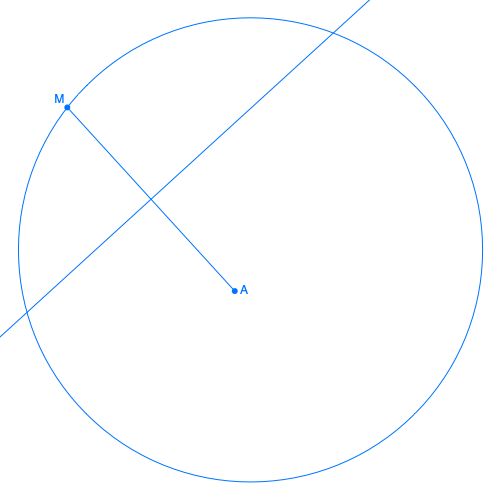Let A be a point inside a circle C
Let M be a point of circle C and D be the line segment bisector of [AM].
Show that wherever M is, D is tangent to an ellipse. Give the focal and axis of the ellipse.
2.